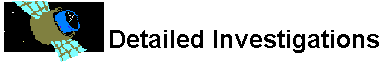# Propulsion

## How do you calculate specific impulse?

To calculate the specific impulse, we first need to calculate the exhaust velocity. Since the real exhaust velocity is exceeding complex to calculate, we will be using some simplifying assumptions to make a simpler equation. Assume that the exhaust velocity follows the following formula:

Ve2 = kRgasTc [1 - (pe/pc)(k-1)/k] / (k-1)

where

k = ratio of specific heats, cp/cv
pe = nozzle exit pressure
pc = combustion chamber pressure
Tc = combustion chamber temperature
Rgas = exhaust flow specific gas constant RR/MM
RR = universal gas constant
MM = exhaust gas molecular weight

Note that k is typically between 1.21-1.26 for a wide range of fuels and oxidizers. Also note that the subscripts (e, c) stand for exit and chamber. Therefore, pc stands for temperature of chamber and pe stands for pressure of exit.

Because we are just approximating, we can simplify the above equation. The second half of the equation:

[1 - (pe/pc)(k-1)/k] / (k-1)

typically ends up as a small number which we will approximate with 1. Since anything multplied by one is itself then a rough guess of a specific impulse is:

Ve2 = kRgasTc

and since k is typically between 1.21-1.26 then we will also remove that term and approximate it as 1 giving us:

Ve2 = RgasTc

This result gives us some interesting observations:

• to get a high exhaust velocity and therefore a high isp we want both a high temperature in the chamber (Tc) and a large exhaust flow gas constant (Rgas)
• for a given temperature (Tc), because Rgas is RR/MM, then if we reduce the molar weight of the exhaust gas then we get a higher velocity.

This then tells us that for better performance (high exhaust velocity), we want fuel-oxidizer mixtures that burn very hot and have a low molar mass exhaust.

The specific impulse is:

Isp = ueq/ge

where

Isp = specific impulse
ueq = total impulse / mass of expelled propellant
ge = acceleration at Earth's surface (9.8 m/s2)

And since we are approximating the speed of a gas with a constant velocity; the momentum of the escaping gas is:

p = mv

where

p = momentum (kg m/s)
m = mass (kg)
v = velocity (m/s)

Notice how masses cancel out and therefore we the Isp is just the velocity of the exhaust gas (Ve) divided by the gravitational attraction of the Earth (ge).Why ion propulsion?How do conventional rockets work?What is thrust?What are the types of rocket propulsion?Why is mass important?What is chemical propulsion?What is specific impulse?What are some rocket propellants?How do you calculate rocket engine performance?